Multiplication of Monomials by Binomials and Trinomials

Chapter 8 Class 8 Algebraic Expressions and Identities
Concept wise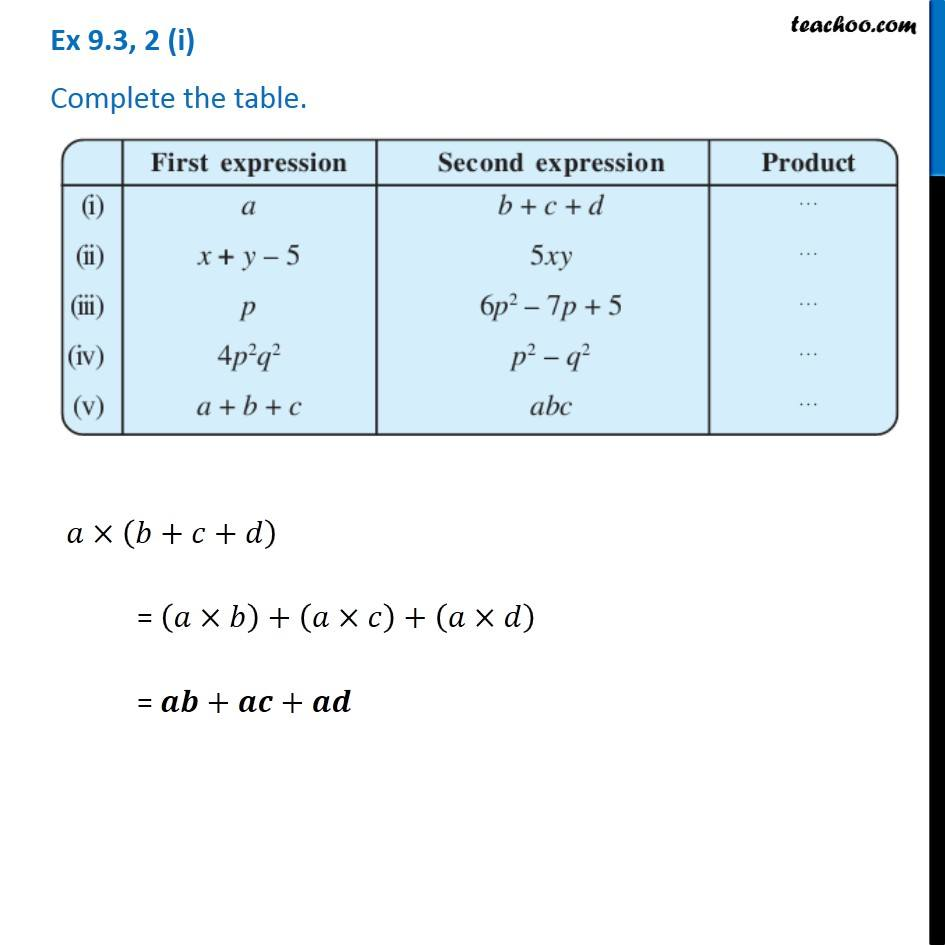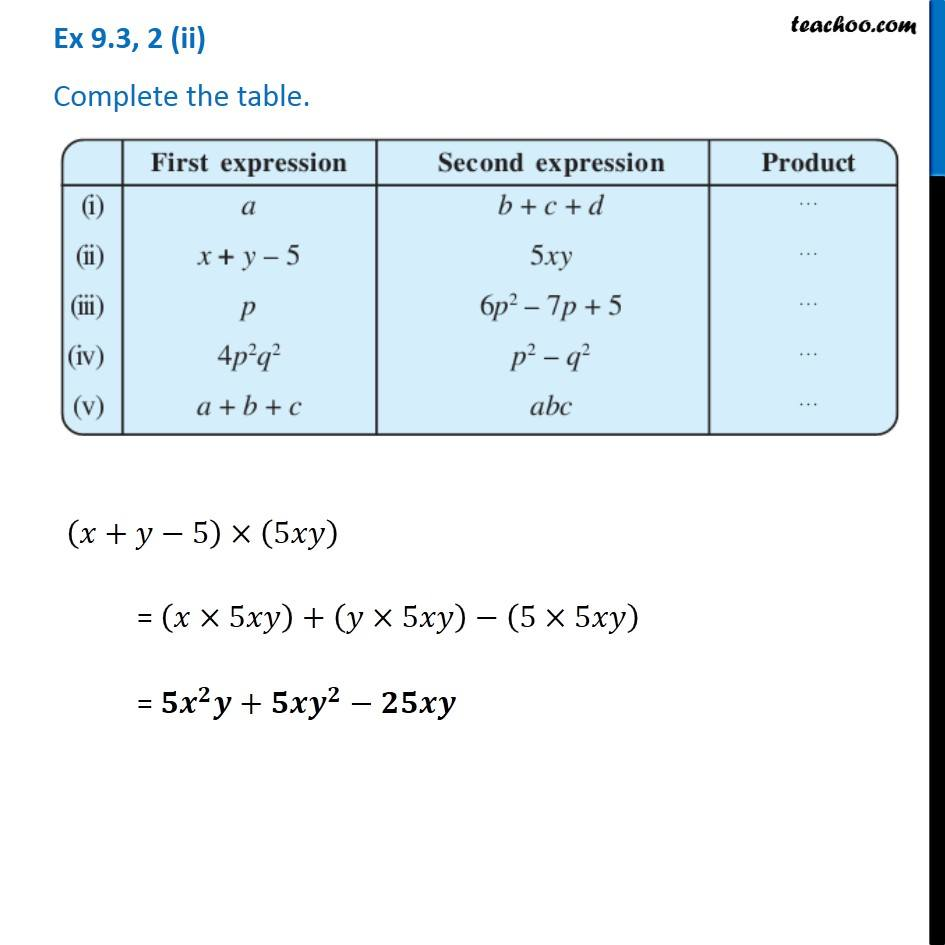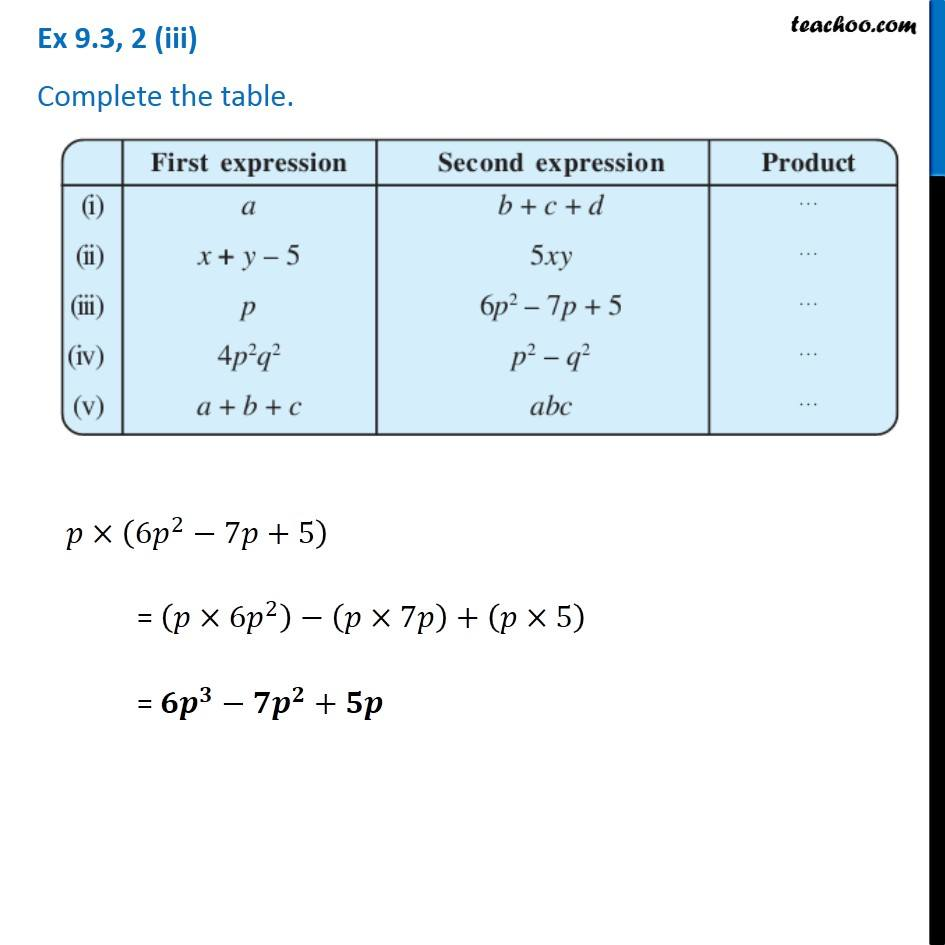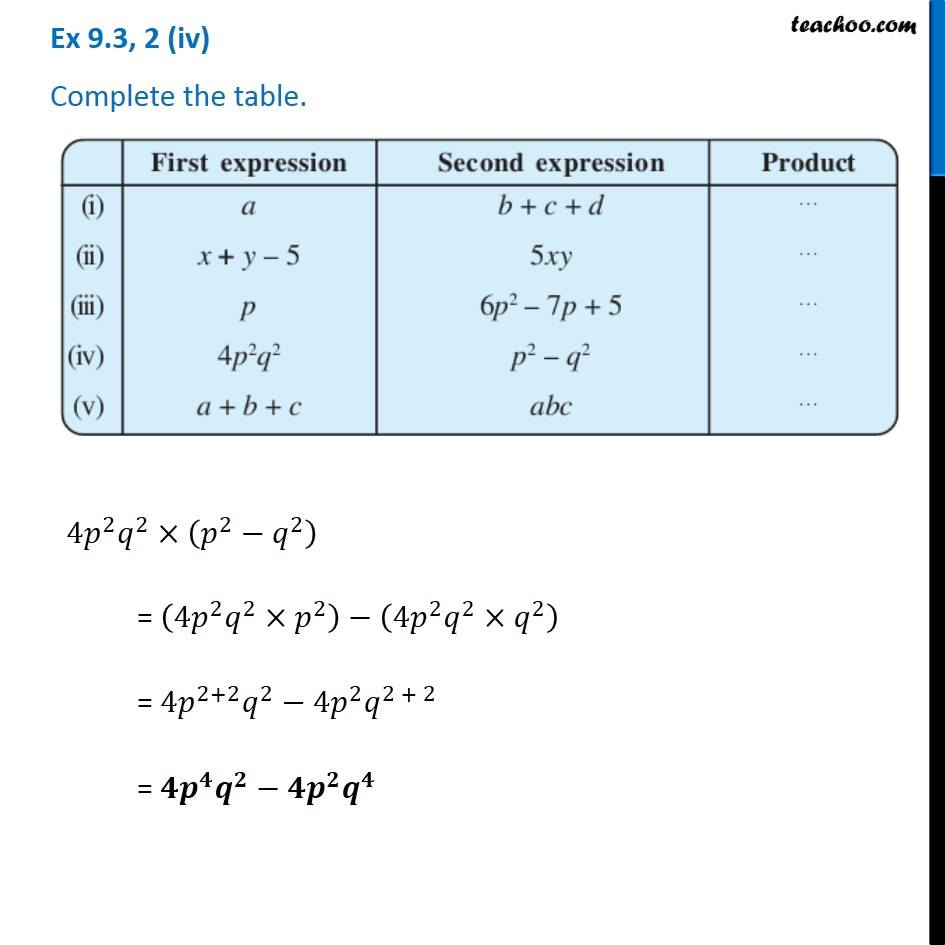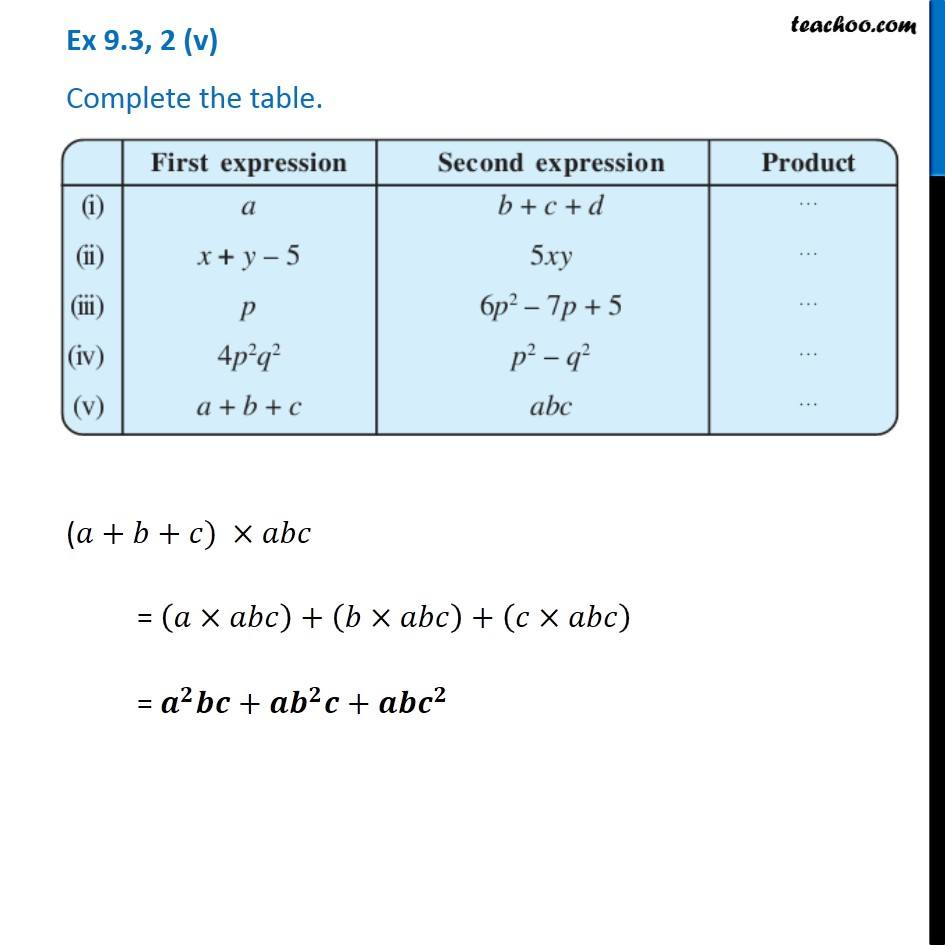Learn in your speed, with individual attention - Teachoo Maths 1-on-1 Class

### Transcript

Ex 8.3, 2 (i) Complete the table. 𝑎×(𝑏+𝑐+𝑑) = (𝑎×𝑏)+(𝑎×𝑐)+(𝑎×𝑑) = 𝒂𝒃+𝒂𝒄+𝒂𝒅 Ex 8.3, 2 (ii) Complete the table. (𝑥+𝑦−5)×(5𝑥𝑦) = (𝑥×5𝑥𝑦)+(𝑦×5𝑥𝑦)−(5×5𝑥𝑦) = 𝟓𝒙^𝟐 𝒚+𝟓𝒙𝒚^𝟐−𝟐𝟓𝒙𝒚 Ex 8.3, 2 (iii) Complete the table. 𝑝×(6𝑝^2−7𝑝+5) = (𝑝×6𝑝^2 )−(𝑝×7𝑝)+(𝑝×5) = 𝟔𝒑^𝟑−𝟕𝒑^𝟐+𝟓𝒑 Ex 8.3, 2 (iv) Complete the table. 4𝑝^2 𝑞^2×(𝑝^2−𝑞^2 ) = (4𝑝^2 𝑞^2×𝑝^2 )−(4𝑝^2 𝑞^2×𝑞^2 ) = 4𝑝^(2+2) 𝑞^2− 4𝑝^2 𝑞^(2 + 2) = 𝟒𝒑^𝟒 𝒒^𝟐−𝟒𝒑^𝟐 𝒒^𝟒 Ex 8.3, 2 (v) Complete the table. (𝑎+𝑏+𝑐) ×𝑎𝑏𝑐 = (𝑎×𝑎𝑏𝑐)+(𝑏×𝑎𝑏𝑐)+(𝑐×𝑎𝑏𝑐) = 𝒂^𝟐 𝒃𝒄+𝒂𝒃^𝟐 𝒄+𝒂𝒃𝒄^𝟐